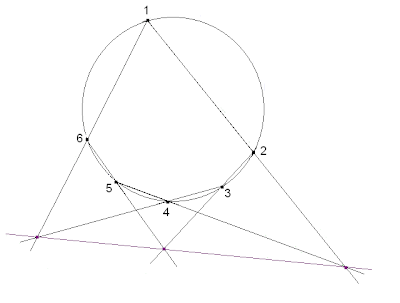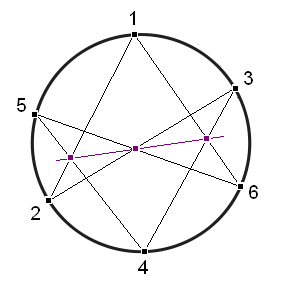## Pages

### Hexagrammum Mysticum Theorem

The mathematician Pascal was most famous for Pascal's triangle, a number pattern that used in the binomial expansion formula. Today, we will introduce a theorem in geometry that bears his name. The Pascal's Hexagrammum Mysticum Theorem states that if we draw a hexagon inscribed in a circle then the three pairs of opposite sides of the hexagon intersect at three points which lie on a straight line.Pascal discovered this amazing hexagon theorem when he was only 16 years old and published it in a poster with the title "Essay pour les coniques" (Essay on Conics). Below is a picture of a rare copy kept in the National Library of France.We can recognize Pascal's hexagon theorem on the figure appeared on the top of the poster. The hexagon is $PQVONK$. The first pair of opposite sides, $PK$ and $VO$, intersect at the point $M$. The second pair of opposite sides, $KN$ and $QV$, intersect at the point $S$. Therefore, by Pascal's theorem, the third pair of opposite sides, $PQ$ and $NO$, must intersect at a point on the line $MS$. In other words, the three lines $MS$, $NO$ and $PQ$ must intersect at a common point.An equivalent statement of Pascal's theorem: three lines $MS$, $NO$, $PQ$ must intersect at a single point

Different configurations of Pascal's theorem

Pascal's theorem has many configurations. The six vertices of the hexagon can be arranged on the circle in any particular order. With each arrangement of the vertices, we have an instance of the Pascal's theorem. Thanks to this variety, Pascal deduced hundreds of corollaries from this theorem.

Now you are invited to draw different pictures of Pascal's theorem. You can draw a circle and then pick out six points: $1$, $2$, $3$, $4$, $5$, $6$, on the circle of any particular order. Draw the intersections of three pairs of lines $\{12, 45\}$, $\{23, 56\}$, $\{34, 61\}$, and then connect the three intersecting points. After that you can choose your own favorite picture of Pascal's theorem.

Here is an example:My favorite picture of Pascal's theorem is the following.Pascal's theorem for the conics

So far, we have only stated the Pascal's theorem for the circle. What makes Pascal's theorem so interesting is that it also holds for all types of conics.  So instead of choosing six points on a circle, we can choose six points on an ellipse, or on a parabola, or on a hyperbola, the theorem is still true! The three intersecting points still lie on a straight line! How amazing is that!

Here is a picture of an ellipse:Here is a parabola:And here is a hyperbola:When the conic degenerated into two straight lines, Pascal's theorem becomes Pappus' Theorem. This is the logo of Math Garden Blog.Let us stop here for now, hope to see you again in the next post.

Homework.

1. Draw different configurations for Pascal's theorem.

2. Find a proof of Pascal's theorem.